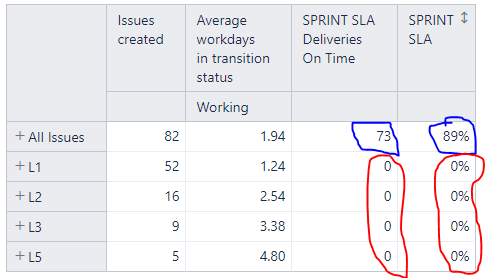# Can´t sum values for aggregated members

Hi there!

I’m having a hard time trying to make my calculated members count the values of itens bellow them, what happens with the non calculated member ( ALL ISSUES ), see bellow:After some research this is my approach for “SPRINT SLA Deliveries On Time”:

CASE WHEN [Issue].CurrentHierarchyMember.Level.Name = “Issue” THEN
Count(
Filter(
Descendants([Issue].CurrentHierarchyMember,[Issue].[Issue]), --goes through all issues
DateCompare([Measures].[Issue resolution date], [Measures].[Fix Verions Release Date]) <= 0
)
)
ELSE
Val(Sum(
Filter(
Descendants([Issue].CurrentHierarchyMember,[Issue].[Issue]), --goes through all issues
DateCompare([Measures].[Issue resolution date], [Measures].[Fix Verions Release Date]) <= 0
)
)
)
END

And this is how i calculate “Fix Verions Release Date”:

[Fix Version].[Version].getMemberByKey(
[Issue].CurrentHierarchyMember.get(‘Fix version IDs’)
).Get(‘Release date’)

Thanks!

You are using the function Descendants to iterate through issues. This function does not work with calculated members. Therefore, you do not have any results there.

I would suggest using a different formula to access issues resolved till the selected version release date:

``````CASE WHEN
DateInPeriod(
[Measures].[Version release date],
[Time].CurrentHierarchyMember
)
THEN
Sum(
PreviousPeriods(
[Time].CurrentHierarchy.Levels("Day").DateMember(
[Measures].[Version release date]).NextMember),
[Measures].[Issues resolved]
)
END
``````

Here is how it looks in the report:

I would suggest avoiding Issue calculated members whenever possible. They would slow down reports and might not work as planned with many formulas, like the one you used.

Daina / support@eazybi.com